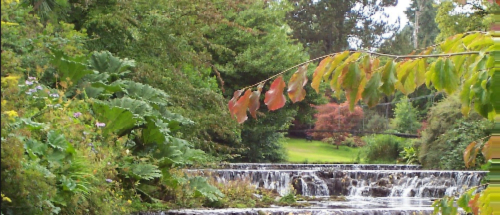# GLSL Digital NoiseWant to add random digital noise to your rendering in GLSL. The following fragment shader will add random noise that changes over time. Full disclosure, I know I started this code from someone’s existing code, but I cannot determine where it’s originally from.

It mimics the weird sort of stepped-offset that you get with digital signals. Here’s a .mkv of the results:

Read more to see the code and how it works.

```
.wp-block-code {
border: 0;
}

.wp-block-code > div {
overflow: auto;
}

.hljs {
box-sizing: border-box;
}

.hljs.shcb-code-table {
display: table;
width: 100%;
}

.hljs.shcb-code-table > .shcb-loc {
color: inherit;
display: table-row;
width: 100%;
}

.hljs.shcb-code-table .shcb-loc > span {
display: table-cell;
}

.wp-block-code code.hljs:not(.shcb-wrap-lines) {
white-space: pre;
}

.wp-block-code code.hljs.shcb-wrap-lines {
white-space: pre-wrap;
}

.hljs.shcb-line-numbers {
border-spacing: 0;
counter-reset: line;
}

.hljs.shcb-line-numbers > .shcb-loc {
counter-increment: line;
}

.hljs.shcb-line-numbers .shcb-loc > span {
}

.hljs.shcb-line-numbers .shcb-loc::before {
border-right: 1px solid #ddd;
content: counter(line);
display: table-cell;
text-align: right;
-webkit-user-select: none;
-moz-user-select: none;
-ms-user-select: none;
user-select: none;
white-space: nowrap;
width: 1%;
}
```#version 330

precision highp float;

layout(location=0) out vec4 frag_colour;

varying vec2 texelCoords;

uniform sampler2D uTexture;

uniform bool uNoise = false;
uniform float uNoiseRange; // 10
uniform float uNoiseIntensity; // 0.0008
uniform float uNoiseTime;

float rand(vec2 co)
{
return fract(sin(dot(co.xy ,vec2(12.9898,78.233))) * 43758.5453);
}

void main()
{
vec2 uv = texelCoords;

if(uNoise)
{
float uvY = uv.y;
uvY *= uNoiseRange;
uvY = float(int(uvY)) * (1.0 / uNoiseRange);
float noise = rand(vec2(uNoiseTime * 0.00001, uvY));
uv.x += noise * uNoiseIntensity;
}

frag_colour = texture(uTexture,uv);
}
``````

Let’s walk through it.

## Noise Uniforms

Aside from the texture uniform that you’d normally expect, you’ll need a few new ones for noise.

``````uniform bool uNoise = false;
uniform float uNoiseRange; //100
uniform float uNoiseIntensity; //0.0008
uniform float uNoiseTime;``````

`uNoise` will determine whether or not the effect is turned on.

`uNoiseRange` will determine how granular the noise actually is.

`uNoiseIntensity` is how powerful the noise is.

`uNoiseTime` is how many milliseconds have passed in total. This value should constantly increase while the effect is on. If it doesn’t increase, the noise won’t animate. You could already have this as a parameter if you’re using an existing game engine.

## Random Function

This is useful in lots of ways, generates a somewhat random value based on the input. If the same input is used again, the same output is given. That determination is often very useful.

``````float rand(vec2 co)
{
return fract(sin(dot(co.xy ,vec2(12.9898,78.233))) * 43758.5453);
}``````

## Applying the Noise

The part of the code that does all the work is only a couple of lines long:

``````if(uNoise)
{
float uvY = uv.y;
uvY *= uNoiseRange;
uvY = float(int(uvY)) * (1.0 / uNoiseRange);
float noise = rand(vec2(uNoiseTime * 0.00001, uvY));
uv.x += noise * uNoiseIntensity;
}``````

Let’s step through it. Firstly, if `uNoise` is false we’re not applying noise. So the first thing we do is change the UV (texture) coordinates by the quality of the noise:

``````uvY *= uNoiseRange;
uvY = float(int(uvY)) * (1.0 / uNoiseRange);``````

This is a little funny, but we’re essentially multiplying the coordinates by the `uNoiseRange` scalar, and then truncating the result by casting it to a vector of `int`s. We finally multiply/divide back down toward the original value.

For example, if the UV coordinates were `0.2, 0.1` then it would:

1. Multiply by `uNoiseRange`, which we can say `98.33f` is a good value.
2. Resulting in `19.066, 9.833`
3. This is truncated to an `int` to result in `19, 9`
4. Which is then multiplied by `1.0 / uNoiseRange`, which is `~0.0101...` giving us a new `vec2` of `~0.19322, ~0.09152`

This modified coordinate is then used as input to our random function which we are going to use to modify the UV coordinate we will actually use for texturing.

``````float noise = rand(vec2(uNoiseTime * 0.00001, uvY));
uv.x += noise * uNoiseIntensity;``````

These are modified by the `uNoiseTime` and `uNoiseIntensity` respectively.

The result is that the UV’s x coordinate is moved slightly to the left or right based on whether or not it’s y coordinate is in the range to be shifted. Modifying `uNoiseRange` will increase or decrease the number of steps or segments to the noise.

For example, here’s a small intensity with a range of `10`.

Then here’s another with the range bumped up to `50`.

And again, but set way down to `2`.

I hope this helps someone add a bit of digital noise to their game. I plan on writing about some additional effects that you can combine with this one to make it looks even better.